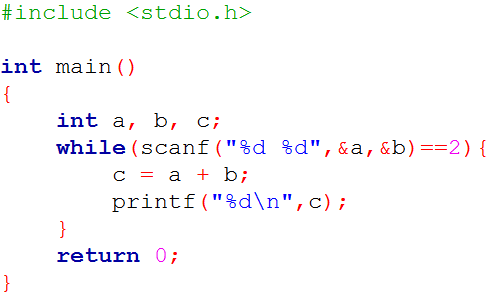Problems
10003 - Add numbers

Add numbers

Time Limit: 1 sec

The Problem

Rakib has started learning programming. He knows the very basic things. But now, he wants to write a program to add two numbers. So, he is trying a lot to find out a hint from the internet. At last, he finds an exact solution like below:Now write a program that adds two numbers.

The Input

The input file consists of several lines. Each line contains 2 integer numbers; A (0<A<1000) and B (0<B<1000). Input is terminated by EOF.

The Output

For each line of input, print the summation of A and B in separate line.

Sample Input

10  20

100  50

Sample Output

30

150

[School & College Level]

Inter School & College Programming Contest (ISCPC) 2015

Problem Setter: Hasnayn Ahmed Shakil